Students get through the TN Board 12th Chemistry Important Questions Chapter 2 p-Block Elements – I which is useful for their exam preparation.

## TN State Board 12th Chemistry Important Questions Chapter 2 p-Block Elements – I

Question 1.
Briefs outline the electronioconfiguration and oxidation state of block elements.

1. They have the general electronic configuration ns2, np1-6.
2. The elements of group 18 have completely filled ‘p’ orbitals and hence more stable than rest of the elements in the block.
3. They show variable oxidation state. The lower oxidation state corresponds to the loss of all the electrons and the higher oxidation state corresponds to the loss of both ‘s’ and ‘p’ electrons.
4. Coming down the group, the stability of the lower oxidation state increase and that of the higher oxidation state increases due to the inert pair effect.
5. Halogens have high electrons affinity values and have show -1 oxidation state.

Question 2.
Explain how does metallic character of ‘p’ block elements very down the group.
Metallic character depends on the tendency to lose an electron by the metal. The magnitude of ionisation energy decides whether an elements is a metal or not. Lower the value of ionisation energy, greater is the electropositive or matellic character. As the ionisation energy decreases down the group, metallic character increases down the group.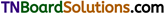Question 3.
What is a metalloid? Name the metalloids present among ‘p’ block elements.
Metalloids are those elements which possess properties intermediate between the metals and non metals. Boron is group 13, silicon and germanium in group 14, arsenic and antimony in group 15, and tellurium in group 16 are metalloids.

Question 4.
The ionisations enthalpy decreases from Boron to aluminium, but from aluminium to thallium only a marginal increase is observed. Mention the cause for this observation.
This is due to the presence of inner d and f-electrons which has poor shielding effect compared to s and p-electrons. As a result, the effective nuclear charge on the valance electrons increases. The attraction between the nucleus and valence electron increases. This results in marginal increase in IE from Al to Ga.

Question 5.
Account for the trend in ionisation enthalpy of group 15/16/18 elefments.
In these groups, the ionisation enthalpy decreases, as we move down the group. Here poor shielding effect of d- and f-electrons are overcome by the increased shielding effect of the additional p-electrons.

Question 6.
How does electronegativity vary from boron to thallium?
The electronegativity first decreases from boron to aluminium and their show a marginal increase. This is due to the fact the increase in the nuclear charge is compensated by the poor shielding effect of ‘d’ and ‘p’ electrons which results in small increase in atomic size.

Question 7.
Mention the causes for the anomalous behaviour of the first element in each group.
The anomalous behaviour is due to its

1. small size
2. high electonegativity and ionisation enthalpy and
3. non-availibity of ‘d’ orbital to expand the valence beyond form.Question 8.
The first member of each group differs from rest of the members in their properties. Explain the statement with an example.
The reason for this behaviour is due to its small size, high electronegativity and non availablity of ‘d’ orbitals for bond formations.
For example, in group 14 elements, the carbon has a tendency for catenation. Except silicon to some extent, this tendency is not shown by germanium, tin and lead. In nitrogen group, nitrogen is a diatomic gas while the rest its members are solids. Among the halogens, fluorine is the strongest oxidising agent.

Question 9.
Explain the term inert pair effect with a suitable example.
‘p’ block elements generally exhibit two oxidation states. The lower oxidation state is obtained by the loss of ‘np’ electrons and the higher oxidation state is obtained by the loss of both ‘ns’ and ‘np’ electrons. For example, corbon family elements exhibit +2 and +4 oxidation state.
As we come down the group, the stability of lower oxidation state increases while that of higher oxidation state decreases. For example,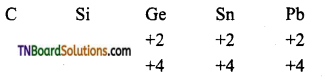i.e., Pb+2 is more stable than Pb+4
Sn+2 is more stable than Sn+4.
This means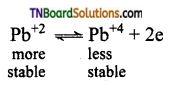PbCl4 is less stable than PbCl2. Hence PbCl4 (Pb+4 ions is a good oxidising agent).
The reason for the inert pair effect is with the increase in nuclear charge, the two electrons in ‘ns’ orbital are firmly bound to the nucleus and becomes ‘inert’ towards bond formation.

Question 10.
Name the allotropes of (i) phosphorus, (ii) tin, (iii) carbon, (iv) silicon, (v) sulphur.

 Element Allotrops (i) phosphorus White phosphorus, Red phosphorus, Scarlet phosphorus, violet and black phosphorus. (ii) carbon Diamond, graphite graphene, fullerenes carbon nanotubes. (iii) tin Grey tin, white tin, rhombic tin, sigma tin. (iv) silicon Amorphous and crystalline silicon. (v) Sulphur Rhombus sulphur and monoclinic sulphur.Question 11.
Briefly detail the trend in physical and chemical properties of boron family.

1. They have the general electronic configuration of ns2 np1.
2. The atomic radius decreases from boron to aluminium due to the increase in nuclear charge and then show a marginal increase from aluminium to thallium. This is due to the screening effect of the ‘d’ and ‘f’ electrons which outweigh the increase in nuclear charge.
3. Down the group, the elements Ga, In and Tl sho w inert pair effect, i.e., The stability of +1 oxidation states increases while that of +3 oxidation state decreases.
4. Boron is a non-metal, while the rest are metals. Their compounds are electrons deficient and act as lewis acids (electron-pair acceptors).
5. Boron differs from the rest of its members in its properties due to its small size, high electronegativity and absence of ‘cf orbitals.
6. All the elements form hydrides, chlorides, oxides, and nitrides.
7. The oxides of boron and aluminium are amphoteric, while the rest are basic.

Question 12.
Give the chemical equation for the following reactions.

1. Boron combines with chromium at 1500K.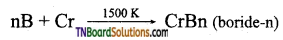2. Boron trichloride reacts with tungsten in the presence of hydrogen at 1500K gaseous.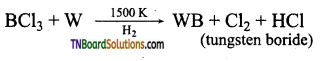3. Boron trifluoride is treated with sodium hydride at 450K.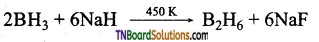4. Boron and chlorine are Ideated.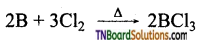5. Boron and nitrogen are heated at high temperatures.
2B + N2 → 2BN

Question 13.
Give one method of preparation of boric anhydride.
Boron is heated with oxygen at 900K. Boric anhydride is formed.Question 14.
How does boron react with (i) conc.H2SO4 and (ii) conc.HNO3 give equations.
Boron being a non-metal is oxidised by these oxidising agents to its oxy acid boric and (H3BO3).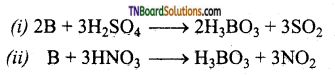Question 15.
How is sodium borate formed from boron? Give equation.
Sodium borate is formed when boron is fused with sodium hydroxide.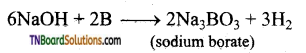Question 16.
Mention the uses of boron.

1. Boron has the capacity to absorb neutrons. Hence, its isotope $$10_{\mathrm{B}_{5}}$$ is used as moderator in nuclear reactors.
2. Amorphous boron is used as a rocket fuel igniter.
3. Boron is essential for the cell walls of plants.
4. Compounds of boron have many applications. For example, eye drops, antiseptics, washing powders etc., contains boric acid and borax. In the manufacture of Pyrex glass, the boric oxide is used.

Question 17.
How is borax prepared from colemanite? Give equation.
The aqueous solution of colemanite (Ca2B6O11) is boiled with sodium carbonate.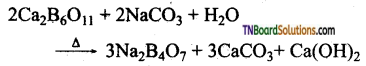Question 18.
All aqueous solution of borax is alkaline in nature. Explain.
The aqueous solution of borax is alkaline due to hydrolysis. Along with boric acid, a strong base NaOH is formed. The pH of the resulting solution is greater than 7. Hence the aqueous solution of borax is alkaline.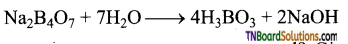Question 19.
What happens when borax is heated? Give equations.
On heating, it first loses its water of crystallisation and on further heating given transparent sodium metaborate (NaBO2).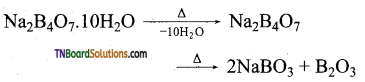Question 20.
Give the equation for the reaction of an aqueous solution of borax with (i) HCl and (ii) H2SO4.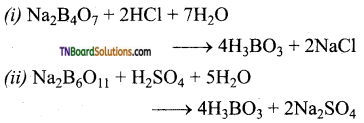Question 21.
Explain how boric acid behaves as a monobasic acid?
Monobasic acids usually release a proton. But boric acid accepts a hydroxyl group instead of donating the proton.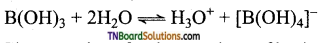Question 22.
Give equations for the reactions of boric acid with sodium hydroxide.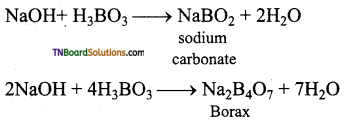Question 23.
What happens when boric acid is heated? Give equations.
On heating, the 373K, boric acid gives metaboric acid and at 413K, it gives tetraboric acid. When heated at red hot, it gives a glassy mass of boric anhydride.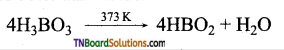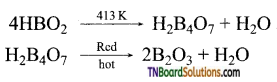Question 24.
What happens when
(i) Boric acid is heated with calcium fluoride in the presence of conc. H2SO4.
(ii) Heated with soda ash? Give equations.
(i) When boric acid is heated with calcium fluoride in the presence of cone. H2SO4 boron trifluoride is formed.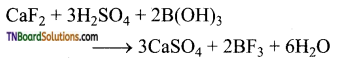(ii) When heated with sodium carbonate (soda ash), it gives borax.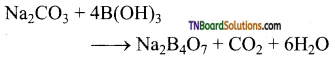Question 25.
Give a brief account of the structure of boric acid.
Boric acid has a two-dimensional layered structure. It consists of [BO2]3- unit and these are linked to each other by hydrogen bonds.Question 26.
Mention the uses of boric acid.

1. Boric acid is used in the manufacture of pottery glazes, glass, enamels and pigments.
2. It is used as an antiseptic and as an eye lotion.
3. It is also used as a food preservative.

Question 27.
Give two methods of preparation of diborane.

1. By the reaction of iodine with sodium borohydride in of diglyme (a solvent).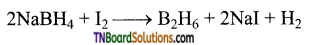2. Heating magnesium boride with HC1 a mixture of volatile boranes is obtained.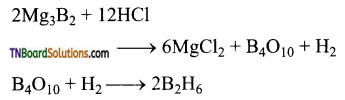Question 28.
Give equations for the reactions between diborane with (i) oxygen (ii) LiH (iii) NH3.

1. Pure diborane does not react with air or O2 at room temperature. But impure diborane gives B2O3. The reaction is exothermic.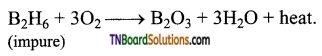2. When treated with lithium hydride, it gives lithium borohydride.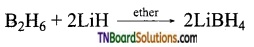3. When treated with excess ammonia, at low temperatures, it gives diborane diammonate. On further heating it gives borazole.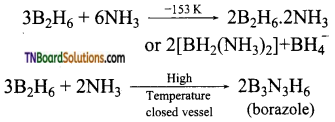Question 29.
What is inorganic benzene? How is it prepared? Write its structure.
Borazole is called inorganic benzene.
Preparation of borazole:
When treated with excess ammonia, at low temperatures, it gives diborane diammonate. On further heating it gives borazole.Structure of borazole: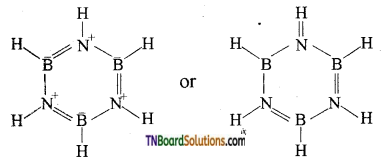Question 30.
Mention the uses of diborane.

1. Diborane is used as a high energy fuel for propellant.
2. It is used as a reducing agent in organic chemistry.
3. It is used in welding torches.

Question 31.
How is boron trifluoride prepared? Give equations.
(i) By heating boron trioxide with calcium fluoride in the presence of cone. H2SO4.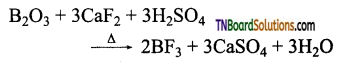(ii) By treating boron trioxide with carbon and fluorine.
B2O3 + 3C+ 3F2 → 2BF3 + 3CO
(iii) By thermal decomposition of benzene diazonium tetrafluoroborate.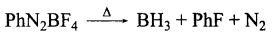Question 32.
Boron trifluoride is a lewis acid. Explain.
Boron trifluoride is an electron-deficient compound. It accepts a pair of electrons from donor atoms such as nitrogens in NH3 and forms a coordinate covalent bond.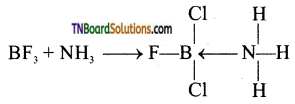Question 33.
Discuss the structure of boron trichloride.
Boron in boron trichloride is sp2 hybridised. The three sp2 hybrid orbitals orient in space in such a way they make an angle of 120°. Each of the sp2 hybrid orbitals contains are unpaired electron. These orbitals overlap with chlorine atoms containing one unpaired electron and form a σ bond. Thus BCl3 is a planar molecule.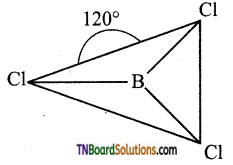Question 34.
How do you convert boron trifluoride to fluoroboric acid? Give equations.
Boron trifluoride, on hydrolysis, gives boric acid. This on treatment with hydrogen fluoride formed gives fluoro boric acid (HBF4).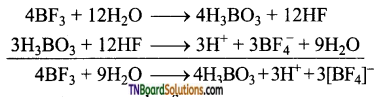Question 35.
Give two methods of preparation of aluminium chloride.

1. Aluminium when treated with hydrochloric acid, aluminium chloride is formed.
2Al + 6HCl → 2AlCl3 + 3H2
2. Aluminium hydroxide, gives aluminium chloride on treatment with dilute hydrochloric acid.
Al(OH)3 + 3HCl → AlCl3 + 3H20

Question 36.
How is aluminium chloride prepared by McAfee process?
Aluminium chloride is obtained by heating a mixture of alumina and coke in a current of chlorine.
Al2O3 + 3C + 3Cl2 → 2AlCl3 + 3CO2
On an industrial scale, it is prepared by chlorinating aluminium around 1000 K.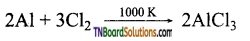Question 37.
Explain why an aqueous solution of aluminium chloride is acidic?
An aqueous solution of aluminium chloride is acidic due to hydrolysis. A strong acid HCl is produced. The resultant solution has a pH less than 7. Hence it is acidic.
AlCl3 + 3H2O → Al(OH)3 + 3HCl

Question 38.
Complete and balance the following equations.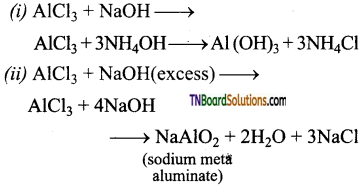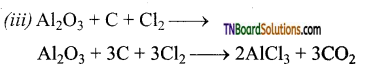Question 39.
Mention the uses of aluminium chloride.

1. Anhydrous aluminium chloride is used as a catalyst in Friedel’s Crafts reactions.
2. It is used for the manufacture of petrol by cracking the mineral oils.
3. It is used as a catalyst in the manufacture of dyes, drugs and perfumes.

Question 40.
What are alums? Give examples.
Alums are double salts of potassium sulphate and aluminium sulphate. It has a general molecular formula M’2SO4.M”2(SO4)3. 24H2O
eg:
Potash alum: K2SO4. Al2(SO4)3. 24H2O
Sodium alum: Na2SO4. Al2(SO4)3. 24H2O
Ammonium alum: (NH4)2SO4. Al2(SO4)3. 24H2O
Chrome alum: K2SO4. Cr2(SO4)3. 24H2OQuestion 41.
Mention the uses of alum.

1. It is used for the purification of water.
2. It is also used for waterproofing and textiles.
3. It is used in dyeing, paper and leather tanning industries.
4. It is employed as a styptic agent to arrest bleeding.

Question 42.
Give a brief account of the trends in properties of carbon group elements.

1. Carbon group elements have the general electronic configuration ns2 np2.
2. The atomic radius increases from carbon to silicon due to the increase in nuclear charge and the added electron enters to next higher energy level than carbon. Hence attraction between the nucleus and the added electron decrease. This accounts for the increase in atomic radius from carbon to silicon. There is a marginal increase in the atomic radius from silicon to tin. This is because even though there is an increase in nuclear charge, the shielding effect of inner ‘d’ and ‘f’ electrons increases. Both these factors are responsible for a small increase in the atomic radii. The decrease in atomic radius from tin to lead, due to an increase in the shielding effect of inner electrons, counterbalances the increase in nuclear charge.
3. A similar trend is followed in the case of the ionisation enthalpy of these elements.
4. Carbon is non-metal, white silicon and germanium are metalloids and tin and lead are metals.
5. Carbon has a greater tendency for catenation than the other elements of these groups. The catenation property decreases down the group.
6. All these elements exhibit +2 and +4 oxidation states. The +2 state becomes more stable down the group than the +4 state due to the inert pair effect.
7. Carbon, silicon, germanium and tin exhibit allotropy.
8. All these elements form oxides. The acidic character of the oxides decreases while that of the basic character increases down the group.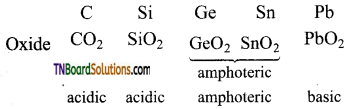9. They form tetrahalides CCl4 is not readily hydrolysed, while the silicon tetrachloride can be readily hydrolysed. The tetrachlorides of other elements of this group are ionic.

Question 43.
Give a brief account of the allotropes of carbon with specific reference to their uses.
Carbon exists in different allotropic forms viz, graphite, diamond, fullerene, carbon nanotubes and graphene.

1. Graphite is soft and is a good conductor of electricity.
2. Diamond is hard and is used for sharpening hard tools, cutting glasses, making bones, and rock drilling.
3. Carbon nanotubes find many applications in nanoscale electrontes, catelysis, polymers and medicine.Question 44.
Explain the structure of graphite.
Graphite has two-dimensional sheet-like structure. Each sheet consists of a hexagonal net of sp2 hybridised carbon of carbon atom with C—C bond distance closer to the C—C bond distance in benzene. Each carbon atom, by making use of its sp2 hybrid orbitals forms three covalent bonds with the neighbouring carbon atom. The fourth electron present in the unhybridized ‘p’ orbital of each carbon atom forms a π bond. The n electrons are delocalised over the entire sheet. This accounts for its electrical conductivity. The sheets are held together by weak vander waals forces and can be readily cleaned. This accounts for its lubricant properly.

Question 45.
Briefly explain the structure of the diamond.
The carbon atoms in diamond are sp3 hybridised and bonded to four neighbouring carbon atoms by σ bonds. This results in a tetrahedral arrangement around each carbon atom that extends to a three-dimensional space lattice. All four valance electrons of carbon are involved in bonding and there is no free electrons and have to diamond is a non-conductor of electricity.

Question 46.
Write a short note on fullerenes.
Fullerenes are newly synthesised allotropes of carbon. These allotropes are discrete molecules such as C32, C50, C60, C70, C76 etc. These molecules have cage-like structures. The C60 molecules have a soccer ball-like structure and are called buckminsterfullerene or buckyballs. It has a fused ring structure consists of 20 six-membered rings and 12 five-membered rings. Each carbon atom is sp2 hybridised and forms three σ bonds & a delocalised π bond giving aromatic character to these molecules.

Question 47.
How is carbon monoxide produced on an industrial scale?
By the reaction of carbon with air. The carbon monoxide formed will contain nitrogen gas also and the mixture of nitrogen and carbon monoxide is called producer gas.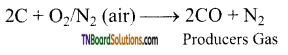The producer gas is then passed through a solution of copper(I) chloride under pressure which results in the formation of CuCl(CO).2H2O. At reduced pressures, this solution releases pure carbon monoxide.Question 48.
Complete the following equations.
(i) HCOOH + H2SO4
(ii) CO + Fe2O3
(iii) CO + H2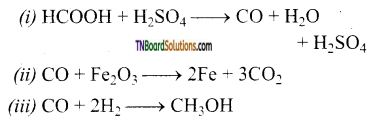Question 49.
Give the equation for the preparation of propanal by oxo process.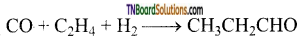Question 50.
What are carbonyls? Give examples.
Carbonyl is a compound that contains carbon monoxide as a ligand. It is a complex compound formed by a transition metal and carbon monoxide. The transition metal in carbonyls exists in a zero oxidation state.
eg: Nickel tetra carbonyl Ni(CO)4
Iron pentacarbonyl Fe(CO)5
Chromium hexacarbonyl Cr(CO)6.

Question 51.
Mention the uses of carbon monoxide.

1. An equimolar mixture of hydrogen and carbon monoxide – ‘water gas and the mixture of carbon monoxide and nitrogen – producer gas is important industrial fuels.
2. Carbon monoxide is a good reducing agent and can reduce many metal oxides to metals.
3. Carbon monoxide is an important ligand and forms a carbonyl compound with transition metals.

Question 52.
How is carbon dioxide produced industrially?
It is produced by burning coke in excess of air.
2CO + O2 → 2CO2

Question 53.
Give an equation for the preparation of carbon dioxide in the laboratory.
CaCO3 + 2HCl → CaCl2 + H2O + CO2Question 54.
Give a brief account of the properties of carbon dioxide.

1. It is an acidic oxide. It produces carbonic acid with water.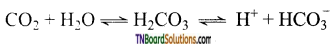2. At high temperatures, it acts as a strong reducing agent. It is reduced to carbon by metals like Mg.
CO2 + Mg → 2MgO + C
3. It is forms water gas with hydrogen.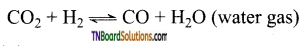4. At temperatures above 3100 K, it undergoes decomposition.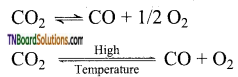Question 55.
Mention the uses of carbon dioxide.

1. Carbon dioxide is used to produce an inert atmosphere for chemical processing.
2. Biologically, it is important for photosynthesis.
3. It is also used as a fire extinguisher and as propellant gas.
4. It is used in the production of carbonated beverages and in the production of foam.

Question 56.
Give equations for the preparation of silicon tetrachloride from (i) silica and (ii) silicon.

1. SiCl4 from SiO2:
SiO2 + 2C + 2Cl2 → SiCl4 + 2CO
2. SiCl4 from Si:
Si + 4HCl → SiCl4 + 2H2

Question 57.
What is the actions of moisture on silicon tetrachloride? Give equations.
In moist air, silicon tetrachloride is hydrolysed to give silica and hydrochloric acid.
SiCl4 + 4H2O → 4HCl + Si(OH)4Question 58.
What is the action of moist ether on silicon tetrachloride?
Silicon tetrachloride is hydrolysed to produce linear perchloro siloxanes. They have the repeating unit. [Cl.(SiCl2O)n.SiCl3 where n = 1 to 6]

Question 59.
Explain the terms alcoholysis and ammonolysis taking silicon tetrachloride as an example.
Alcoholysis:
The chlorine in silicon tetrachloride can be substituted by nucleophiles such as OH, OR, etc., using suitable reagents. For example, it forms silicic esters with alcohols.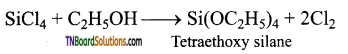Ammonialysis:
Similarly silicon tetrachloride undergoes ammonialysis to form chlorosilazanes.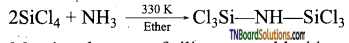Question 60.
Mention the uses of silicon tetrachloride.

1. Silicon tetrachloride is used in the production of semiconducting silicon.
2. It is used as a starting material in the synthesis of silica gel, silicic esters, a binder for ceramic materials.

Question 61.
(i) What are silicones?
(ii) Mention the various types of silicons?
(iii) Give the various properties of silicones.
(i)

1. Silicones or polysiloxanes are organosilicon polymers with general empirical formula (R2SiO).
2. These silicones may be linear or cross-linked polymers.
3. Linear polymers contain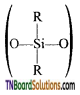as a repeating unit.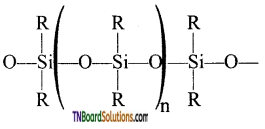4. Complex cross-linked polymers have the structure.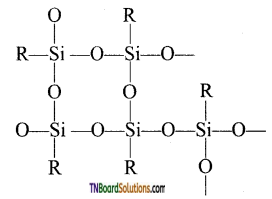(ii) Types of silicones: The following are the types of silicones.

1. Linear silicones
2. Cyclic silicones

(iii) Properties of silicones:

1. All silicones are water repellant.
2. They are thermal and electrical insulators.
3. Chemically they are inert.Question 62.
Explain the formation of straight-chain or linear silicones.
The straight-chain or linear silicone is formed by the hydrolysis of dialkyl dichloro silanes.
(R2SiCl2 where R is an alkyl group).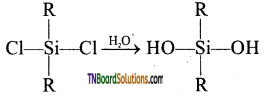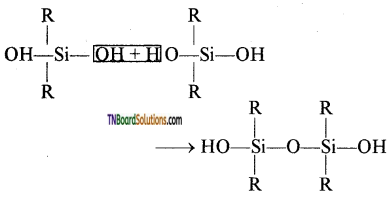This compound further reacts with another molecule of R2Si(OH)2 and form linear dialkyl chloro silanes.

Question 63.
Explain the formation of a complex cross-linked polymer with a suitable example.
The hydrolysis of mono alkylchloro silanes gives a complex cross-linked polymer.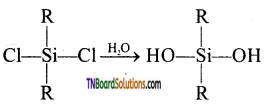When all the ‘OH’ groups are removed as water molecules, acyclic or ring silicones are obtained.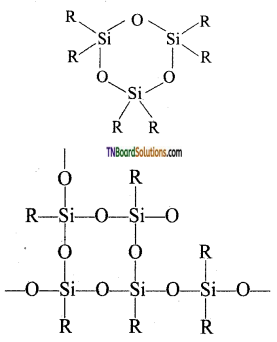Question 64.
What are synthetic rubber and synthetic resins?
Synthetic rubber is silicones that are bridged together by methylene (—CH2—) or similar groups. Synthetic resins are obtained by blending silicones with organic resins such as acrylic esters.Question 65.
What are silicates? Give examples for various types of silicates.
Silicates are minerals that contain [SiO4]-4 tetrahedra units linked together in different patterns.
Examples for other silicates: Phenacite BeSiO4 Olivine (Fe/Mg)2 SiO4.
Examples for phyllosilicates: Thortveitite Sc2Si2O7
Examples for cyclic silicates: Beryl [Bl3Al2(SiO3)6]
Examples for chain silicate(pyroxenes) spodumene: LiAl(SiO3)2
Example for double chain silicates or amphiboles: Asbestos.
Examples for sheet silicates: Talc, mica etc. Examples for three-dimensional silicates: Quartz, feldspar, zeolites etc.

Question 66.
What are ortho silicates?
Ortho silicates contain discrete [SiO4]-4 tetrahedral units. The silicon atom is at the centre of the tetrahedra and the four oxygen atoms occupy the comers of the tetrahedra.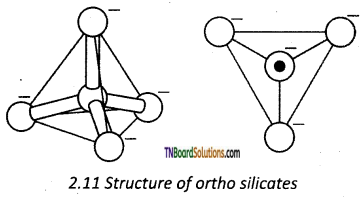In phenacite Be2SiO4, Be+2 ions are tetrahedrally surrounded by O-2 ions.

Question 67.
What are pyrosilicates? How are they formed?
Pyrosilicates are silicates containing [Si2O7]-2 units. They are formed by joining two [SiO4]-4 tetrahedral units by sharing one oxygen atom.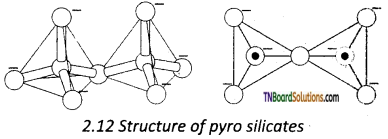Question 68.
What are cyclic silicates? How are they formed?
Silicates that contain (SiO3)n2n- ions which are formed by linking three or more tetrahedral SiO44- units cyclically are called cyclic silicates. Each silicate unit shares two of its oxygen atoms with other units.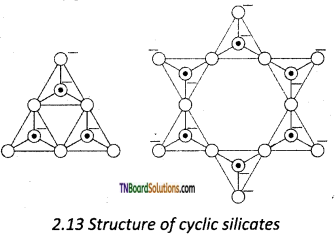Question 69.
How are pyroxenes formed? [OR]
Explain the formation of chain silicates.
These silicates contain [(SiO3)n]2n- ions ‘ formed by linking ‘n’ number of tetrahedral [SiO4]4- units linearly. Each silicate unit shares two of its oxygen atoms with other units.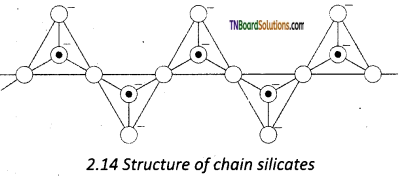Question 70.
Explain the formation of sheet or phyllo silicates.
Silicates that contain (Si2O5)n2n- are called sheet or phyllo silicates. In these, Each [SiO4]4- tetrahedron unit shares three oxygen atoms with others and thus by forming two-dimensional sheets. These sheets of silicates form layered structures in which silicate sheets are stacked over each other. The attractive forces between these layers are very week, hence they can be cleaved easily just like graphite.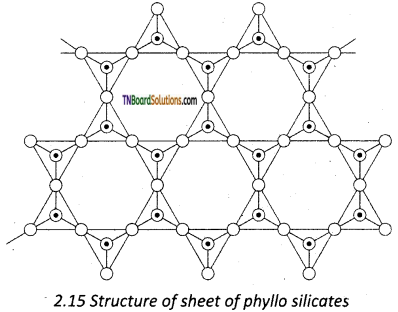Question 71.
What are amphiboles? How are they formed?
Amphiboles are double-chain silicates that contain (Si4O11)n6n- ions. There are two types,

1. These sharing 3 vertices and
2. Those sharing only 2 vertices. Examples: asbestos.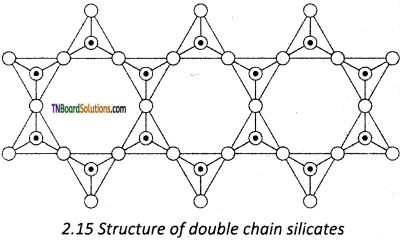Question 72.
Briefly explain the structure of three-dimensional silicates.
Silicates in which all the oxygen atoms of [SiO4]4- tetrahedra are shared with other tetrahedra to form a three-dimensional network are called three dimensional or tecto silicates. They have general formula (SiO2)n.
eg: Quartz These tecto silicates can be converted into Three-dimensional aluminosilicates by replacing [SiO4]4- units by [AlO4]5- units. E.g. Feldspar, Zeolites etc.,

1. Among the following pairs of elements which act as semiconductors?
(a) C and Si
(b) Si and Ge
(c) B and Al
(d) B and Si
(b) Si and Ge2. Among ‘p’ block elements which group show +6 oxidations state?
(a) Icosagens
(b) Tetragens
(c) Prictogens
(d) chalcogens
(d) chalcogens
Hint: Chalcogens are group 16 elements which has a general electronic configuration ns2np4. The loss of all ns and np electrons results in +6 oxidation state.

3. Choose the correct statement:
(a) There is an increase in ionisation energy down the group as a result.
(b) All the elements in group 13 are metals.
(c) Boron and silicon exhibit diagonal relationship.
(d) Boron trifluoride is readily hydrolysed.
(c) Boron and silicon exhibit diagonal relationship.

4. Aluminium (III) chloride is stable where as thallium (III) chloride is highly unstable this is due to:
(a) inert pair effect
(b) increase in metallic character down the group
(c) decrease in metallic character down the group
(d) aluminium chloride is covalent
(a) inert pair effect5. Which of the following is used as a moderator in nuclear reacters?
(a) $${ }_{6} \mathrm{C}^{14}$$
(b) $${ }_{5} \mathrm{B}^{10}$$
(c) $${ }_{5} \mathrm{N}^{14}$$
(d) $${ }_{8} \mathrm{O}^{17}$$
(b) $${ }_{5} \mathrm{B}^{10}$$

6. An aqueous solutions of borox is:
(a) acidic
(b) basic
(c) neutral
(d) is acidic as well as basic
(b) basic
Hint: Borox hydrolysis to give a strong base
Na2B4O7 + 7H2O → 4H3BO3 + 2NaOH

7. Borax Bead test is used to identify:
(a) boron
(c) onetal cations

8. Boric acid is:
(a) a weak mono basic acid
(b) a strong tetrabasic acid
(c) weak mono acidic base
(d) a diabasic acid
(a) a weak mono basic acid

9. Boric acid is heated to red hot. The product obtained is:
(a) metaboric acid
(b) pyroboric acid
(c) Boron trioxide
(d) all
(c) Boron trioxide
Hint: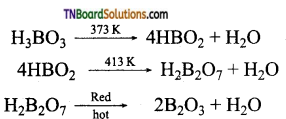10. Choose the incorrect statement with regard to boric acid:
(a) The structure consists of [BO3]-3 units linked to each other by hydrogen bonds.
(b) It is a monobasic acid as it releases a proton.
(c) It is a monobasis acid, as it accepts a hydroxyl ion.
(d) Boric acid is used as an antiseptic.
(b) It is a monobasic acid as it releases a proton.

11. Which is not true with respect to the structure of diborane?
(a) Each boron atoms, are sp3 hybridised
(b) The form B—H bonds are two centre two electron bonds.
(c) The B—H—B bonds are three centre – three electron bonds.
(d) The B—H—B bonds are three centre- two electron bonds.
(c) The B—H—B bonds are three centre – three electron bonds.

12. Producer gas is a mixture of:
(a) carbon monoxide and nitrogen
(b) carbon monoxide and hydrogen
(c) carbon dioxide and nitrogen
(d) carbon dioxide and hydrogen
(a) carbon monoxide and nitrogen

13. The hydrolysis of CH3SiCl3 yields:
(b) cyclic polymer
(c) linear polymer
(d) silicols14. Phenacite is:
(a) Be3SiO4
(b) an orthosilicate
(c) both (a) and (b)
(d) a cyclic silicate